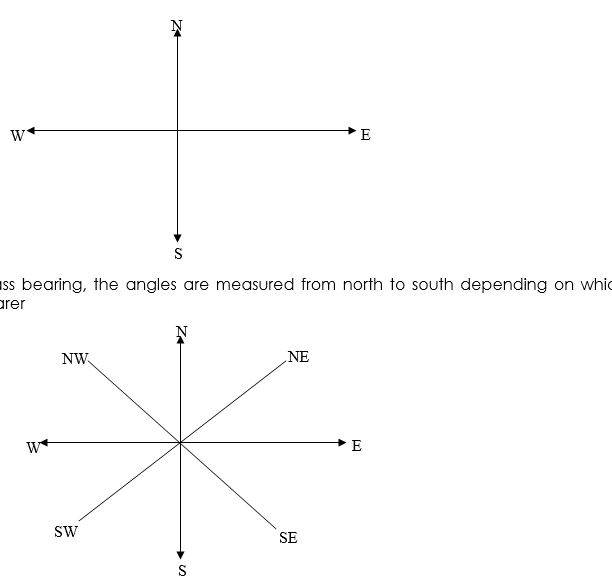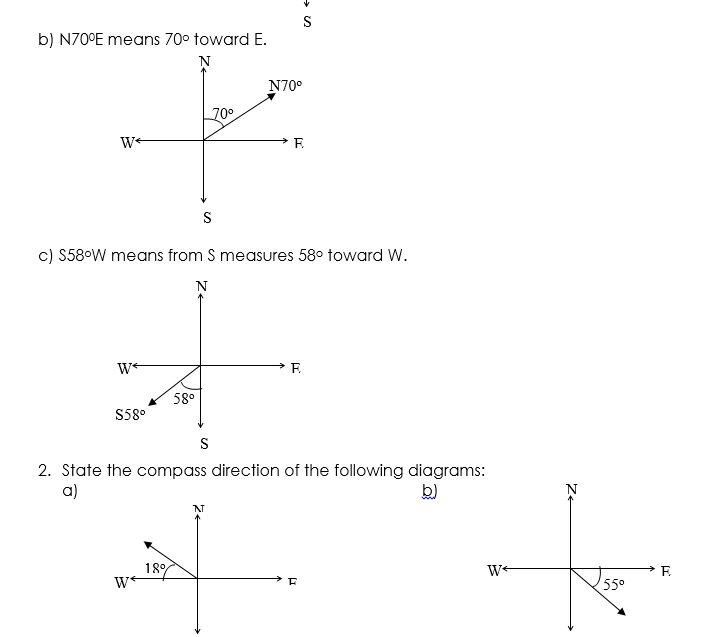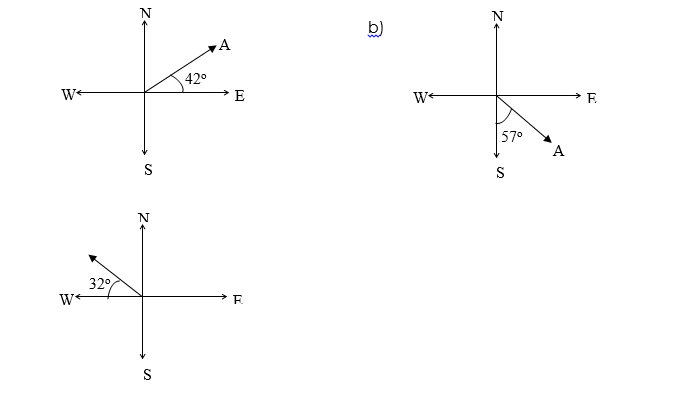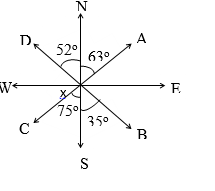# Bearing and Distances

Welcome to Class !!

In today’s Mathematics class, We will be discussing Bearing and Distances. We hope you enjoy the class!CONTENT:

i. Compass bearing

ii Three figure bearing

iii. Finding the bearing of a point from another

### COMPASS BEARING

A bearing gives the direction between two points in terms of an angle in degrees. The two types of bearing are compass bearing and three-figure bearings.

The four major compass directions are North (N) South (S) East (E) and West (W)Apart from the four main points or directions, there are also four main secondary directions i.e. NE (northeast), SE (south-east), SW (south-west), NW (north-west). The angle between each point is 45o

Worked examples

Draw a sketch to show each of these bearings marketing the angles clearly.

a) N35oW    (B) N70oE    (C.) S58oW

Solution

1. N35oW means from N measure 35o toward the W or 35oW of NSolution

In a), the direction start from a wrong point (W) instead of N, therefore,

90 – 18 = 72o

i.e. N72oW

In b), the direction starts from a wrong point (E) instead of S therefore:

90 – 55 = 35o i.e. S35oE

Evaluation: Class Work

Find the compass direction of point A from point O in these diagrams.NGM BK CHAPTER 23, page 185 – 187

Essential Mathematics for JSS BK 2, CHAPTER 24, pg 246-247

##### THREE-FIGURE BEARINGS

Three-figure bearings are given as the number of degrees from north, measured in a clockwise direction. Any direction can be given as a three-figure bearing. Three-digit are always given but angles less than 100o need extra zero to be written in front of the digits e.g. 008o, 060o, 070o up to 099o

Worked Example

Find the three-figure bearings of A, B, C, and D from XSolution

1. The arrow N shows the direction N, NXA = 63o. the bearing of A from X is 063o
2. NXB = 180 – 35 = 145o. The bearing of B from X is 145o
3. NXC clockwise = 180 + 75 = 255o. The bearing of C from X is 255o
4. NXD clockwise = 360 – 52 = 308o. The bearing of D from X is 308o.

Evaluation:

In the figure below, find the bearings of A, B, C and D from X.Reference

NGM Bk. 2 Chapter 23, page 180 – 19

Evaluation

In each diagram below, calculate

i) the bearing of B from A

ii) the bearing of A from BGENERAL EVALUATION

From a point P, the bearing of a house is 060o. From a point Q 100m due east of P, the bearing is 330o.

Draw a labelled sketch to show the positions of P, Q and the house.

REVISION QUESTION

1. A girl is facing East. If she turns clockwise through 2 right angles, then the direction she would be facing is ……………………..
2. A student is facing South-East. If he turns anticlockwise through 1800, then the direction he would be facing is …………………..

WEEKEND ASSIGNMENT

1. The bearing of X from Y is 196o. The bearing of Y from X is 016o       B. 074o      C. 106o     D. 196o
2. A boat sails on a bearing of 225o. Using compass bearing, in what direction is the boat sailing? South East     B. North-East     C. South West     D. North West
3. The bearing of point A from B is 058o. Find the bearing of point B from point 058o     B. 122o     C. 3020     D. 238o
4. Which of the following statements is not true when we specify a direction with bearing? Measure the angle from North   B. Measure anticlockwise     C. Measure clockwise     D. Always use three digits
5. In the diagram below, which of the following angles is the bearing of P from Q? 065o    B. 2450     C. 295o     D. 115oWe have come to the end of this class. We do hope you enjoyed the class?

Should you have any further question, feel free to ask in the comment section below and trust us to respond as soon as possible.

In our next class, we will be talking about Statistics: Data Presentation. We are very much eager to meet you there.

Are you a Parent? Share your quick opinion and win free 2-month Premium Subscription

### 5 thoughts on “Bearing and Distances”

1.I NEED MORE EXAMPLES FOR THREE FIGURE BEARING

2.i need more examples for compass bearing

3.i need help. i am lost

4.Shomoye Mariam

The bearing of sokoto from Kaduna is 050 what Is the bearing of Kaduna from sokoto

1.Deborah Temitope Babalola

If the bearing is less than 180 degrees, add 180. And if it is more than 180, subtract 180 degrees in other to get the back or reciprocal bearing
To your question 050+180 =230. Hence the bearing of Kaduna from sokoto is 230 degrees

Don`t copy text!